Courses

# Huffman Coding Electronics and Communication Engineering (ECE) Notes | EduRev

## Communication Theory

Created by: Machine Experts

## Electronics and Communication Engineering (ECE) : Huffman Coding Electronics and Communication Engineering (ECE) Notes | EduRev

The document Huffman Coding Electronics and Communication Engineering (ECE) Notes | EduRev is a part of the Electronics and Communication Engineering (ECE) Course Communication Theory.
All you need of Electronics and Communication Engineering (ECE) at this link: Electronics and Communication Engineering (ECE)

Huffman  Coding

The Shannon-Fano algorithm doesn't always generate an optimal code. In 1952, David A. Huffman gave a different algorithm that always produces an optimal tree for any given probabilities. While the Shannon-Fano tree is created from the root to the leaves, the Huffman algorithm works from leaves to the root in the opposite direction.

1. Create a leaf node for each symbol and add it to frequency of occurrence.
2. While there is more than one node in the queue:
• Remove the two nodes of lowest probability or frequency from the queue
• Prepend 0 and 1 respectively to any code already assigned to these nodes
• Create a new internal node with these two nodes as children and with probability equal to the sum of the two nodes' probabilities.
• Add the new node to the queu
3. The remaining node is the root node and the tree is complete.

Example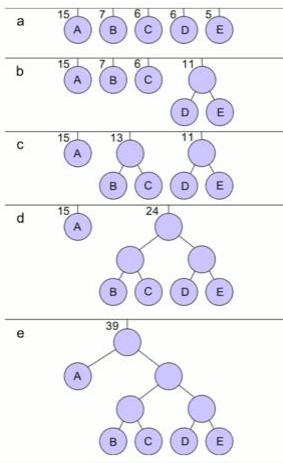Huffman Algorithm

Using the same frequencies as for the Shannon-Fano example above, viz: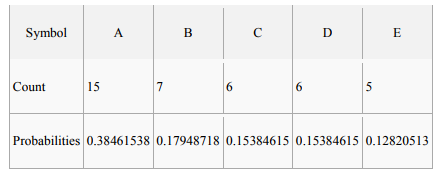In this case D & E have the lowest frequencies and so are allocated 0 and 1 respectively and grouped together with a combined probability of 0.28205128. The lowest pair now are B and C so they're allocated 0 and 1 and grouped together with a combined probability of 0.33333333. This leaves BC and DE now with the lowest probabilities so 0 and 1 are prepended to their codes and they are combined. This then leaves just A and BCDE, which have 0 and 1 prepended respectively and are then combined. This leaves us with a single node and our algorithm is complete

The code lengths for the different characters this time are 1 bit for A and 3 bits for all other characters.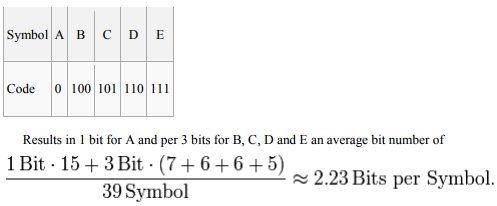Lempel–Ziv–Welch:

Lempel–Ziv–Welch (LZW) is a universal lossless data compression algorithm created by Abraham Lempel, Jacob Ziv, and Terry Welch. It was published by Welch in 1984 as an improved implementation of the LZ78 algorithm published by Lempel and Ziv in 1978. The algorithm is simple to implement and has very high throughput

ALGORITHM:

Idea:

The scenario described in Welch's 1984 paper encodes sequences of 8-bit data as fixed-length 12-bit codes. The codes from 0 to 255 represent 1-character sequences consisting of the corresponding 8-bit character, and the codes 256 through 4095 are created in a dictionary for sequences encountered in the data as it is encoded. At each stage in compression, input bytes are gathered into a sequence until the next character would make a sequence for which there is no code yet in the dictionary. The code for the sequence (without that character) is emitted, and a new code (for the sequence with that character) is added to the dictionary.

The idea was quickly adapted to other situations. In an image based on a color table, for example, the natural character alphabet is the set of color table indexes, and in the 1980s, many images had small color tables (on the order of 16 colors). For such a reduced alphabet, the full 12-bit codes yielded poor compression unless the image was large, so the idea of a variable-width code was introduced: codes typically start one bit wider than the symbols being encoded, and as each code size is used up, the code width increases by 1 bit, up to some prescribed maximum (typically 12 bits).

Further refinements include reserving a code to indicate that the code table should be cleared (a "clear code", typically the first value immediately after the values for the individual alphabet characters), and a code to indicate the end of data (a "stop code", typically one greater than the clear code). The clear code allows the table to be reinitialized after it fills up, which lets the encoding adapt to changing patterns in the input data. Smart encoders can monitor the compression efficiency and clear the table whenever the existing table no longer matches the input well.

Since the codes are added in a manner determined by the data, the decoder mimics building the table as it sees the resulting codes. It is critical that the encoder and decoder agree on which variety of LZW is being used: the size of the alphabet, the maximum code width, whether variable-width encoding is being used, the initial code size, whether to use the clear and stop codes (and what values they have). Most formats that employ LZW build this information into the format specification or provide explicit fields for them in a compression header for the data

Encoding:

A dictionary is initialized to contain the single-character strings corresponding to all the possible input characters (and nothing else except the clear and stop codes if they're being used). The algorithm works by scanning through the input string for successively longer substrings until it finds one that is not in the dictionary. When such a string is found, the index for the string less the last character (i.e., the longest substring that is in the dictionary) is retrieved from the dictionary and sent to output, and the new string (including the last character) is added to the dictionary with the next available code. The last input character is then used as the next starting point to scan for substrings.

In this way, successively longer strings are registered in the dictionary and made available for subsequent encoding as single output values. The algorithm works best on data with repeated patterns, so the initial parts of a message will see little compression. As the message grows, however, the compression ratio tends asymptotically to the maximum.

Decoding:

The decoding algorithm works by reading a value from the encoded input and outputting the corresponding string from the initialized dictionary. At the same time it obtains the next value from the input, and adds to the dictionary the concatenation of the string just output and the first character of the string obtained by decoding the next input value. The decoder then proceeds to the next input value (which was already read in as the "next value" in the previous pass) and repeats the process until there is no more input, at which point the final input value is decoded without any more additions to the dictionary.

In this way the decoder builds up a dictionary which is identical to that used by the encoder, and uses it to decode subsequent input values. Thus the full dictionary does not need be sent with the encoded data; just the initial dictionary containing the single-character strings is sufficient (and is typically defined beforehand within the encoder and decoder rather than being explicitly sent with the encoded data.)

Variable-width codes:

If variable-width codes are being used, the encoder and decoder must be careful to change the width at the same points in the encoded data, or they will disagree about where the boundaries between individual codes fall in the stream. In the standard version, the encoder increases the width from p to p + 1 when a sequence ω + s is encountered that is not in the table (so that a code must be added for it) but the next available code in the table is 2 (the first code requiring p + 1 bits). The encoder emits the code for ω at width p (since that code does not require p + 1 bits), and then increases the code width so that the next code emitted will be p + 1 bits wide. The decoder is always one code behind the encoder in building the table, so when it sees the code for ω, it will generate an entry for code 2p − 1. Since this is the point where the encoder will increase the code width, the decoder must increase the width here as well: at the point where it generates the largest code that will fit in p bits. Unfortunately some early implementations of the encoding algorithm increase the code width and then emit ω at the new width instead of the old width, so that to the decoder it looks like the width changes one code too early. This is called "Early Change"; it caused so much confusion that Adobe now allows both versions in PDF files, but includes an explicit flag in the header of each LZW-compressed stream to indicate whether Early Change is being used. Most graphic file formats do not use Early Change.

When the table is cleared in response to a clear code, both encoder and decoder change the code width after the clear code back to the initial code width, starting with the code immediately following the clear code. Packing order:

Since the codes emitted typically do not fall on byte boundaries, the encoder and decoder must agree on how codes are packed into bytes. The two common methods are LSB-First ("Least Significant Bit First") and MSB-First ("Most Significant Bit First"). In LSB-First packing, the first code is aligned so that the least significant bit of the code falls in the least significant bit of the first stream byte, and if the code has more than 8 bits, the high order bits left over are aligned with the least significant bit of the next byte; further codes are packed with LSB going into the least significant bit not yet used in the current stream byte, proceeding into further bytes as necessary. MSB-first packing aligns the first code so that its most significant bit falls in the MSB of the first stream byte, with overflow aligned with the MSB of the next byte; further codes are written with MSB going into the most significant bit not yet used in the current stream byte.

Example:

The following example illustrates the LZW algorithm in action, showing the status of the output and the dictionary at every stage, both in encoding and decoding the data. This example has been constructed to give reasonable compression on a very short message. In real text data, repetition is generally less pronounced, so longer input streams are typically necessary before the compression builds up efficiency. The plaintext to be encoded (from an alphabet using only the capital letters) is:

The # is a marker used to show that the end of the message has been reached. There are thus 26 symbols in the plaintext alphabet (the 26 capital letters A through Z), plus the stop code #. We arbitrarily assign these the values 1 through 26 for the letters, and 0 for '#'. (Most flavors of LZW would put the stop code after the data alphabet, but nothing in the basic algorithm requires that. The encoder and decoder only have to agree what value it has.)

A computer will render these as strings of bits. Five-bit codes are needed to give sufficient combinations to encompass this set of 27 values. The dictionary is initialized with these 27 values. As the dictionary grows, the codes will need to grow in width to accommodate the additional entries. A 5-bit code gives 25 = 32 possible combinations of bits, so when the 33rd dictionary word is created, the algorithm will have to switch at that point from 5-bit strings to 6-bit strings (for all code values, including those which were previously output with only five bits). Note that since the all-zero code 00000 is used, and is labeled "0", the 33rd dictionary entry will be labeled 32. (Previously generated output is not affected by the code-width change, but once a 6-bit value is generated in the dictionary, it could conceivably be the next code emitted, so the width for subsequent output shifts to 6 bits to accommodate that.)

The initial dictionary, then, will consist of the following entries: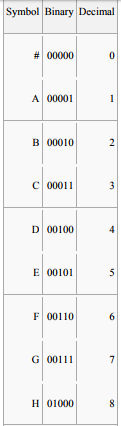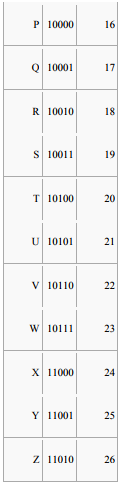Encoding:

Buffer input characters in a sequence ω until ω + next character is not in the dictionary. Emit the code for ω, and add ω + next character to the dictionary. Start buffering again with the next character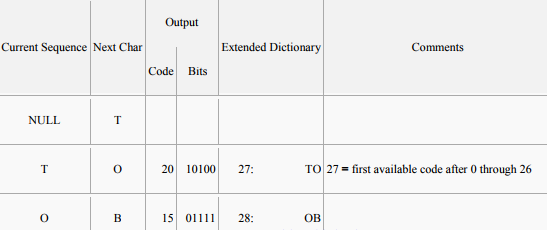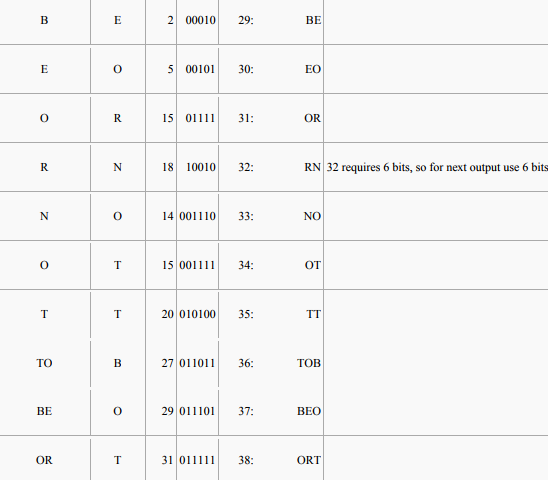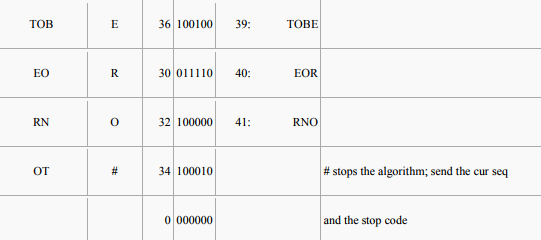Unencoded length = 25 symbols × 5 bits/symbol = 125 bits

Encoded length = (6 codes × 5 bits/code) + (11 codes × 6 bits/code) = 96 bits.

Using LZW has saved 29 bits out of 125, reducing the message by almost 22%. If the message were longer, then the dictionary words would begin to represent longer and longer sections of text, allowing repeated words to be sent very compactly.

Decoding: To decode an LZW-compressed archive, one needs to know in advance the initial dictionary used, but additional entries can be reconstructed as they are always simply concatenations of previous entries.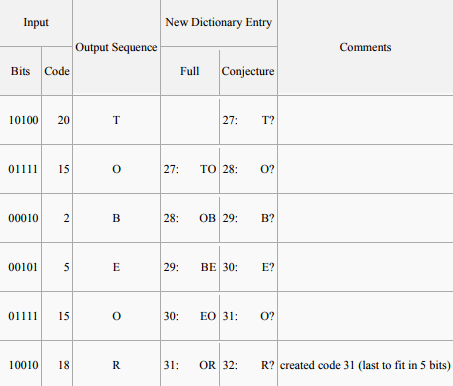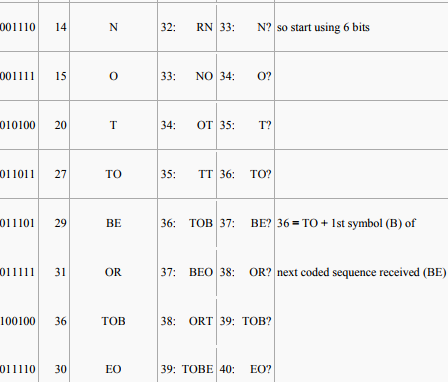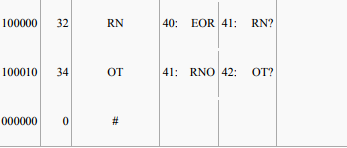At each stage, the decoder receives a code X; it looks X up in the table and outputs the sequence χ it codes, and it conjectures χ + ? as the entry the encoder just added — because the encoder emitted X for χ precisely because χ + ? was not in the table, and the encoder goes ahead and adds it. But what is the missing letter? It is the first letter in the sequence coded by the next code Z that the decoder receives. So the decoder looks up Z, decodes it into the sequence ω and takes the first letter z and tacks it onto the end of χ as the next dictionary entry. This works as long as the codes received are in the decoder's dictionary, so that they can be decoded into sequences. What happens if the decoder receives a code Z that is not yet in its dictionary? Since the decoder is always just one code behind the encoder, Z can be in the encoder's dictionary only if the encoder just generated it, when emitting the previous code X for χ. Thus Z codes some ω that is χ + ?, and the decoder can determine the unknown character as follows:

1. The decoder sees X and then Z.

2. It knows X codes the sequence χ and Z codes some unknown sequence ω.

3. It knows the encoder just added Z to code χ + some unknown character,

4. and it knows that the unknown character is the first letter z of ω.

5. But the first letter of ω (= χ + ?) must then also be the first letter of χ.

6. So ω must be χ + x, where x is the first letter of χ.

7. So the decoder figures out what Z codes even though it's not in the table,

8. and upon receiving Z, the decoder decodes it as χ + x, and adds χ + x to the table as the value of Z.

This situation occurs whenever the encoder encounters input of the form cScSc, where c is a single character, S is a string and cS is already in the dictionary, but cSc is not. The encoder emits the code for cS, putting a new code for cSc into the dictionary. Next it sees cSc in the input (starting at the second c of cScSc) and emits the new code it just inserted. The argument above shows that whenever the decoder receives a code not in its dictionary, the situation must look like this.

Although input of form cScSc might seem unlikely, this pattern is fairly common when the input stream is characterized by significant repetition. In particular, long strings of a single character (which are common in the kinds of images LZW is often used to encode) repeatedly generate patterns of this sort. Further coding:

The simple scheme described above focuses on the LZW algorithm itself. Many applications apply further encoding to the sequence of output symbols. Some package the coded stream as printable characters using some form of Binary-to-text encoding; this will increase the encoded length and decrease the compression frequency. Conversely, increased compression can often be achieved with an adaptive entropy encoder. Such a coder estimates the probability distribution for the value of the next symbol, based on the observed frequencies of values so far. Standard entropy encoding such as Huffman coding or arithmetic coding then uses shorter codes for values with higher probabilities.

Uses:

When it was introduced, LZW compression provided the best compression ratio among all well-known methods available at that time. It became the first widely used universal data compression method on computers. A large English text file can typically be compressed via LZW to about half its original size.

LZW was used in the program compress, which became a more or less standard utility in Unix systems circa 1986. It has since disappeared from many distributions, for both legal and technical reasons, but as of 2008 at least FreeBSD includes both compress and uncompress as a part of the distribution. Several other popular compression utilities also used LZW, or closely related methods.

LZW became very widely used when it became part of the GIF image format in 1987. It may also (optionally) be used in TIFF and PDF files. (Although LZW is available in Adobe Acrobat software, Acrobat by default uses the DEFLATE algorithm for most text and color-table-based image data in PDF files.)

Offer running on EduRev: Apply code STAYHOME200 to get INR 200 off on our premium plan EduRev Infinity!

,

,

,

,

,

,

,

,

,

,

,

,

,

,

,

,

,

,

,

,

,

;